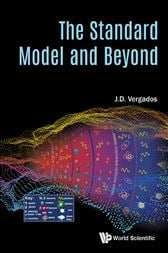# The Standard Model and Beyond£36.20
£32.95
###### ISBNs
• 9789813228559
• 9789813228573
-->

This book contains a systematic and pedagogical exposition of recent developments in particle physics and cosmology. It starts with two introductory chapters on group theory and the Dirac theory. Then it proceeds with the formulation of the Standard Model (SM) of Particle Physics, particle content and symmetries, fully exploiting the material of the first two chapters. It discusses the concept of gauge symmetries and emphasizes their role in particle physics. It then analyses the Higgs mechanism and the spontaneous symmetry breaking (SSB). It explains how the particles (gauge bosons and fermions) after the SSB acquire a mass and get admixed. The various forms of the charged currents are discussed in detail as well as how the parameters of the SM, which cannot be determined by the theory, are fixed by experiment, including the recent LHC data and the Higgs discovery. Quantum chromodynamics is discussed and various low energy approximations to it are presented. The Feynman diagrams are introduced and applied, at the level of first year graduate students.

Examples are the evaluation of the decay widths of the gauge bosons and some cross sections for interesting processes such as Rutherford scattering, electron-proton scattering (elementary proton or described by a form factor, and inelastic scattering) and Compton scattering.

After that the classic topics like the role of C, P, CP symmetries and the experimental methods needed to verify their conservation or violation are discussed in some detail. Topics beyond the standard model, like supersymmetry for pedestrians and grand unification, are discussed.

To this end neutrino oscillations, dark matter and baryon asymmetry are also briefly discussed at the first year graduate level. Finally, the book contains an exhibition of recent developments in cosmology, especially from the elementary particle point of view.

-->Contents:
• Mathematical Prerequisite A: Elements of Group Theory
• Mathematical Prerequisite B: The Dirac Theory
• The Standard Model: Particle Content and Symmetry
• The Higgs Mechanism
• Fermion Masses and Currents
• The SM SU(3) Group; Quantum Chromodynamics
• Rates and Cross Sections in Electroweak Theory
• Supersymmetry for Pedestrians
• Grand Unification; The SU(5) Example
• A Brief Introduction to Cosmology
• Aspects of Neutrino Physics; Neutrino Oscillations
• Discrete Symmetries: C, P, T and All That
• Appendix: Some Elementary Aspects of Particle Physics
-->

• World Scientific Publishing Company; August 2017
• ISBN: 9789813228573